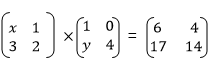Home MATHEMATICS TOPIC 7: MATRICES AND TRANSFORMATION ~ MATHEMATICS FORM 4

# TOPIC 7: MATRICES AND TRANSFORMATION ~ MATHEMATICS FORM 4

379
0
SHAREOperations on Matrices
The Concept of a Matrix
Explain the concept of a matrix
Definition:
A matrix is an array or an Orderly arrangement of objects in rows and columns.
Each object in the matrix is called an element (entity).
Consider the following table showing the number of students in each stream in each form.
 Form I II III IV Stream A 38 35 40 28 Stream B 36 40 34 39 Stream C 40 37 36 35
From the above table, if we enclose the numbers in brackets without changing
their arrangement, then a matrix is farmed, this can be done by
removing the headings and the bracket enclosing the numbers (elements)
and given a name (normally a capital letter).
Nowthe above information can be presented in a matrix form as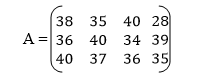Any matrix has rows and columns but sometimes you may find a matrix with only row without Colum or only column without row.
In the matrix A above, the numbers 38, 36 an 40 form the first column and 38, 35, 40 and 28 form the first row.
Matrix A above has three (3) rows and four (4) columns.
In
the matrix A, 34 is the element (entity) in the second row and third
column while 28 lies in the first row and fourth column. The plural form
of matrix is matrices.
Normallymatrices are named by capital letters and their elements by small letters which represent real numbers.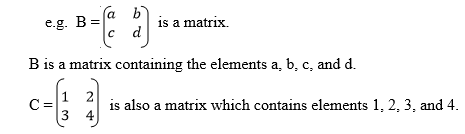Order of a matrix (size of matrix)
The order of a matrix or size of a matrix is given by the number of its rows and the number of its columns.
So if A has m rows and n columns, then the order of matrix is m x n.
It
is important to note that the order of any matrix is given by stating
the number of its rows first and then the number of its columns.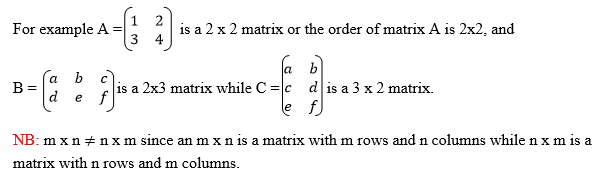Types of matrices:
The following are the common types of matrices:-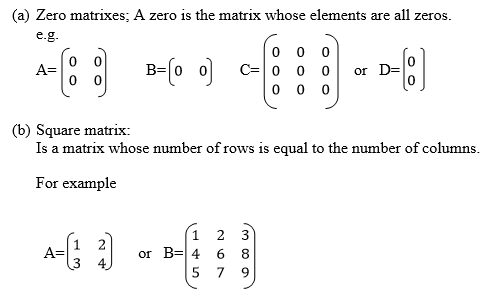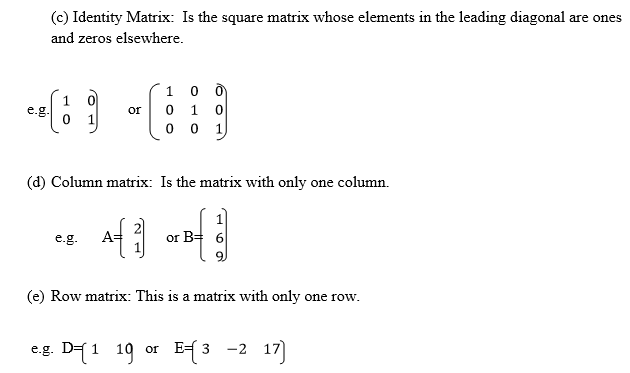Matrices of order up to 2 X 2
Add matrices of order up to 2 X 2
When adding or subtracting one matrix from another, the corresponding elements (entities) are /added or subtracted respectively.
This being the case, we can only perform addition and subtraction of matrices with the same orders.
Example 1
Given that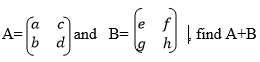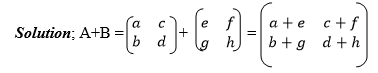Matrices of order up to 2 X 2
Subtract matrices of order up to 2 X 2
Example 2
Given that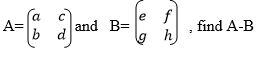Example 3
Solve for x, y and z in the following matrix equation;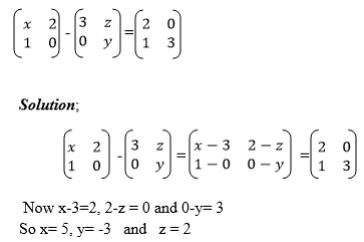Exercise 1
Determine the order of each of the following matrices;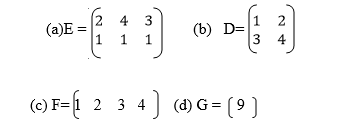2. Given that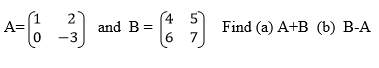3. Given that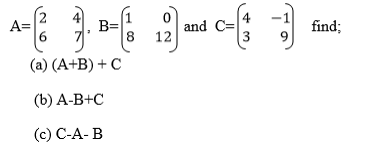4.
A house wife makes the following purchases during one week: Monday 2kg
of meat and loaf of bread Wednesday, 1kg of meat and Saturday, 1kg of
meat and one loaf of bread. The prices are 6000/= per kg of meat and
1. Write a 3×2 matrix of the quantities of items purchased over the three days .
2. Write a 2×1 column matrix of the unit prices of meat and bread.
5. Solve for x, y and z in the equation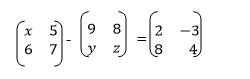If M is any square matrix, that is a matrix with order mxm or nxn and Z is another matrix with the same order as m such that
M+ Z= Z+M = M then Z is the additive identity matrix.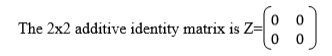The additive inverse of a matrix.
If
A and B are any matrices with the same order such that A+B = Z, then it
means that either A is an additive inverse of B or B is an additive
inverse of A that is B=-A or A= -B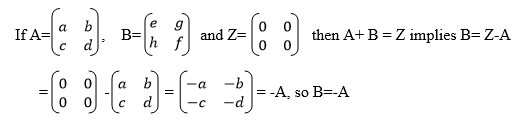Example 4
Find the additive inverse of A,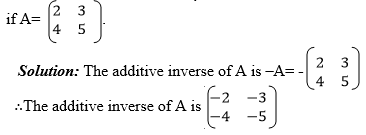Example 5
Find the additive identity of B if B is a 3×3 matrix.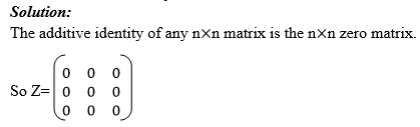A Matrix of Order 2 X 2 by a Scalar
Multiply a matrix of order 2 X 2 by a scalar
A matrix can be multiplied by a constant number (scalar) or by another matrix.
Scalar multiplication of matrices:
Rule: If A is a matrix with elements say a, b, c and d, or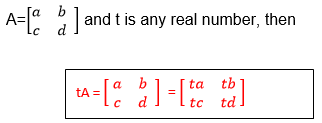Example 6
Given that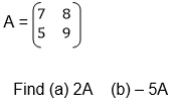Solution;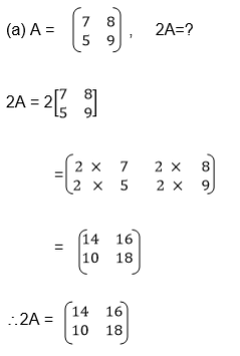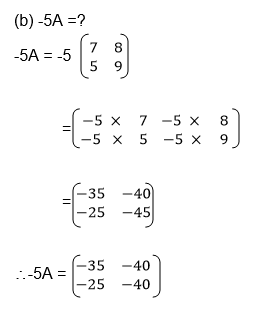Example 7
Given,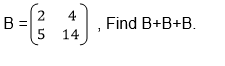Solution;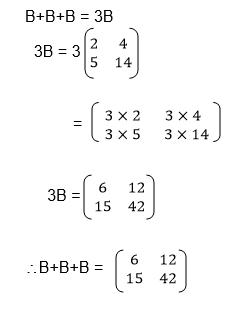Two Matrices of order up to 2 X 2
Multiply two matrices of order up to 2 X 2
Multiplication of Matrix by another matrix: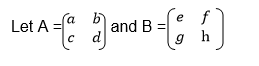AB is the product of matrices A and B while BA is the product of matrix B and A.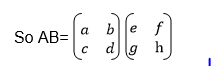In
AB, matrix A is called a pre-multiplier because it comes first while
matrix B is called the post multiplier because it comes after matrix A.
Rules of finding the product of matrices;
1. The pre –multiplier matrix is divided row wise, that is it is divided according to its rows.
2. The post multiplier is divided according to its columns.
3. Multiplication is done by taking an element from the row and multiplied by an element from the column.
4. In
rule (iii) above, the left most element of the row is multiplied by the
top most element of the column and the right most element from the row
is multiplied by the bottom most element of the column and their sums
are taken: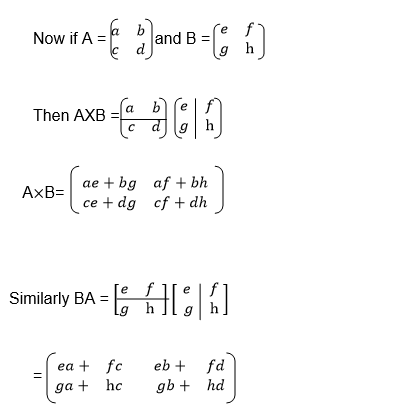Therefore
it can be concluded that matrix by matrix multiplication is only
possible if the number of columns in the pre-multiplier is equal to the
number of rows in the post multiplier.
Example 8
Given That;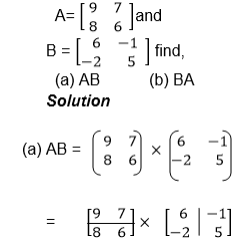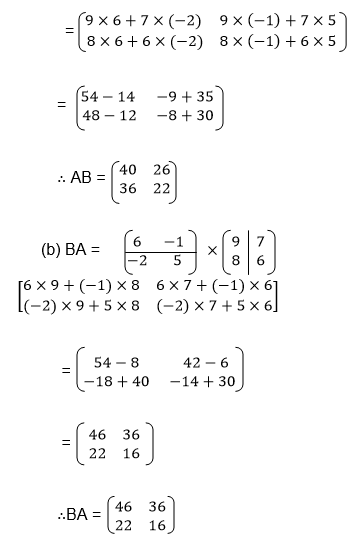From
the above example it can be noted that AB≠BA, therefore matrix by
matrix multiplication does not obey commutative property except when the
multiplication involves and identity matrix i.e. AI=IA=A
Example 9
Let,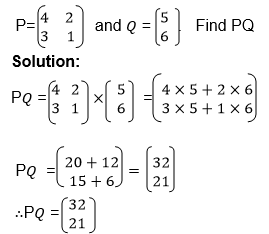Example 10
Find C×D if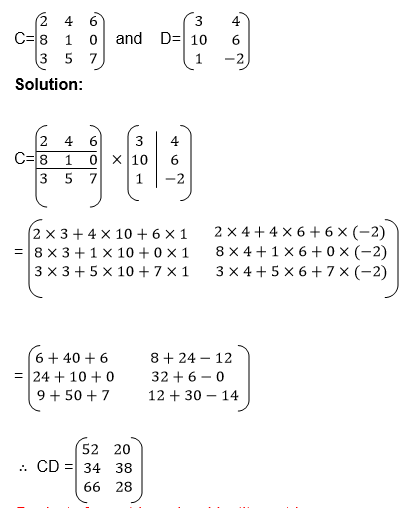Product of a matrix and an identity matrix:
If A is any square matrix and I is an identity matrix with the same order as A, then AI=IA=A
Example 11
Given;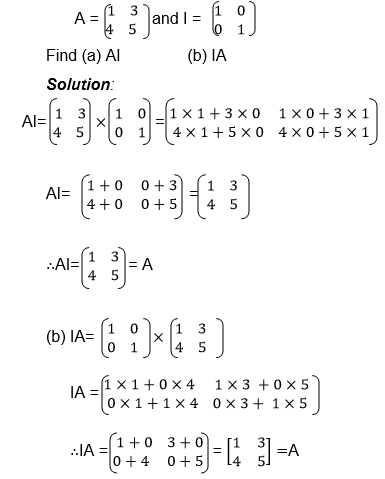Exercise 2
1. Given that A= (3 4) and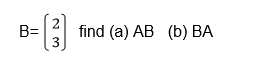2. If,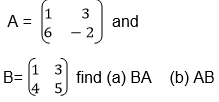3.Using the matrices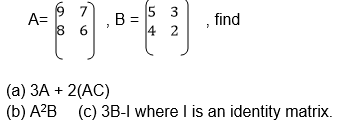4.Find the values of x and y if